# Class 11 Maths NCERT Solutions for Chapter 2 Relations and Functions Miscellaneous Exercise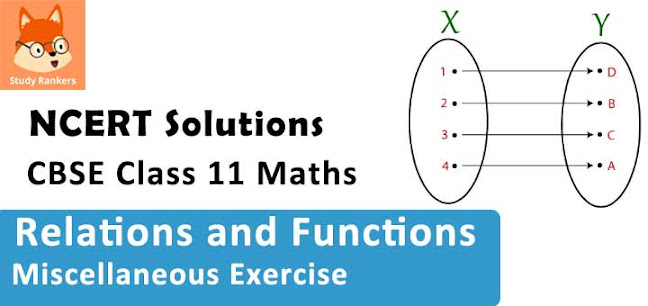### Relations and Functions Miscellaneous Exercise Solutions

1. The relation f is defined by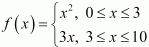The relation g is defined by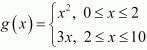Show that f is a function and g is not a function.

Solution

The relation f is defined asIt is observed that for
0 ≤ x < 3, f(x) = x2
3 < x ≤ 10, f(x) = 3x
Also, at x = 3, f(x) = 32 = 9 or f(x) = 3×3 = 9
i.e., at x = 3, f(x) = 9
Therefore, for 0 ≤ x ≤ 10, the images of f(x) are unique.
Thus, the given relation is a function.
The relation g is defined asIt can be observed that for x = 2, g(x) = 22 = 4 and g(x) = 3 × 2 = 6
Hence, element 2 of the domain of the relation g corresponds to two different images i.e., 4 and 6.
Hence, this relation is not a function.

2. If f(x) = x2, find [f(1.1) - f(1)]/(1.1 - 1)

Solution

f(x) = x23. Find the domain of the function f(x) = (x2 + 2x + 1)/(x2 - 8x + 12)

Solution

The given function is f(x) = (x2 + 2x + 1)/(x2 - 8x + 12)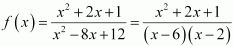It can be seen that function f is defined for all real numbers except at x = 6 and x = 2
Hence, the domain of f is R = {2, 6}.

4. Find the domain and the range of the real function f defined by f(x) = √(x - 1) .

Solution

The given real function is f(x) = √(x - 1) .
It can  be seen that √(x - 1) is defined for (x - 1) ≥ 0.
i.e., f(x) = √(x - 1) is defined for x ≥ 1.
Therefore, the domain of f is the set of all real numbers greater than or equal to 1 i.e., the domain of f = (1, ∞)
As x ≥ 1 ⇒ (x - 1) ≥ 0 ⇒ √(x - 1) ≥ 0
Therefore, the range of f is the set of all real numbers greater than or equal to 0 i.e., the range of f = [0,∞).

5.  Find the domain and the range of the real function f defined by f (x) = |x – 1|.

Solution

The given real function is f (x) = |x – 1|.
It is clear that |x – 1| is defined for all real numbers.
∴Domain of f = R
Also, for x ∈ R, |x – 1| assumes all real numbers.
Hence, the range of f is the set of all non-negative real numbers.

6. Let f = [{x , x2 /(1 + x2 )} : x ∈ R] be a function from R into R. Determine the range of f.

Solution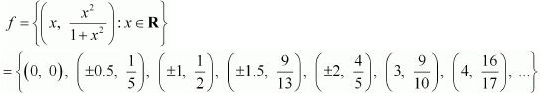The range of f is the set of all second elements. It can be observed that all these elements are greater than or equal to 0 but less than 1.
[Denominator is greater numerator]
Thus, range of f = [0, 1)

7. Let fg: R → R be defined, respectively by f(x) = + 1, g(x) = 2x – 3. Find f + gf – g and f/g.

Solution

fg: R → Ris defined as f(x) = + 1, g(x) = 2x – 3
(f + g) (x) = f(x) + g(x) = (x + 1) + (2x – 3) = 3x – 2
∴(f + g) (x) = 3x – 2
(f – g) (x) = f(x) – g(x) = (x + 1) – (2x – 3) = x + 1 – 2x + 3 = – x + 4
∴ (f – g) (x) = –x + 4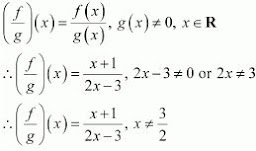8. Let f = {(1, 1), (2, 3), (0, –1), (–1, –3)} be a function from Z to Z defined by f(x) = ax + b, for some integers a, b. Determine a, b.

Solution

f = {(1, 1), (2, 3), (0, –1), (–1, –3)}
f(x) = ax + b
(1, 1) ∈ f
⇒ f(1) = 1
⇒ a × 1 + b = 1
⇒ a + b = 1
(0, –1) ∈ f
⇒ f(0) = –1
⇒ a × 0 + b = –1
⇒ b = –1
On substituting b = –1 in a + b = 1,
we obtain a + (–1) = 1
⇒ a = 1 + 1 = 2.
Thus, the respective values of a and b are 2 and –1.

9. Let R be a relation from N to N defined by R = {(a, b): a, b ∈ N and a = b2}. Are the following true?
(i) (a, a) ∈ R, for all a ∈ N
(ii) (a, b) ∈ R, implies (b, a) ∈ R
(iii) (a, b) ∈ R, (b, c) ∈ R implies (a, c) ∈ R.

Solution

R = {(a, b): a, b ∈ N and a = b2}
(i) It can be seen that 2 ∈ N;however, 2 ≠ 22 = 4.
Therefore, the statement “(a, a) ∈ R, for all a ∈ N” is not true.

(ii) It can be seen that (9, 3) ∈ N because 9, 3 ∈ N and 9 = 32.
Now, 3 ≠ 92 = 81; therefore, (3, 9) ∉ N
Therefore, the statement “(a, b) ∈ R, implies (b, a) ∈ R” is not true.

(iii) It can be seen that (16, 4) ∈ R, (4, 2) ∈ R because 16, 4, 2 ∈ N and 16 = 42 and 4 = 22.
Now, 16 ≠ 22 = 4; therefore, (16, 2) ∉ N
Therefore, the statement “(a, b) ∈ R, (b, c) ∈ R implies (a, c) ∈ R” is not true.

10. Let A = {1, 2, 3, 4}, B = {1, 5, 9, 11, 15, 16} and = {(1, 5), (2, 9), (3, 1), (4, 5), (2, 11)}. Are the following true?
(i) f is a relation from A to B (ii) f is a function from A to B.

Solution

A = {1, 2, 3, 4} and B = {1, 5, 9, 11, 15, 16}
∴A × B = {(1, 1), (1, 5), (1, 9), (1, 11), (1, 15), (1, 16), (2, 1), (2, 5), (2, 9), (2, 11), (2, 15), (2, 16), (3, 1), (3, 5), (3, 9), (3, 11), (3, 15), (3, 16), (4, 1), (4, 5), (4, 9), (4, 11), (4, 15), (4, 16)}
It is given that f = {(1, 5), (2, 9), (3, 1), (4, 5), (2, 11)}

(i) A relation from a non-empty set A to a non-empty set B is a subset of the Cartesian product A ×
B.
It is observed that f is a subset of A × B.
Thus, f is a relation from A to B.

(ii) Since the same first element i.e., 2 corresponds to two different images i.e., 9 and 11, relation f is not a function.

11. Let f be the subset of Z × Z defined by f = {(ab, a + b): a, b ∈ Z}. Is f a function from Z to Z: justify your answer.

Solution

The relation f is defined as f = {(ab, a + b): a, b ∈ Z}
We know that a relation f from a set A to a set B is said to be a function if every element of set A has unique images in set B.
Since 2, 6, –2, –6 ∈ Z, (2 × 6, 2 + 6), (–2 × –6, –2 + (–6)) ∈ f
i.e., (12, 8), (12, –8) ∈ f
It can be seen that the same first element i.e., 12 corresponds to two different images i.e., 8 and –8. Thus, relation f is not a function.

12. Let A = {9, 10, 11, 12, 13} and let f: A → N be defined by f(n) = the highest prime factor of n. Find the range of f.

Solution

A = {9, 10, 11, 12, 13}
f: A → N is defined as
f(n) = The highest prime factor of n
Prime factor of 9 = 3
Prime factors of 10 = 2, 5
Prime factor of 11 = 11
Prime factors of 12 = 2, 3
Prime factor of 13 = 13
∴f(9) = The highest prime factor of 9 = 3
f(10) = The highest prime factor of 10 = 5
f(11) = The highest prime factor of 11 = 11
f(12) = The highest prime factor of 12 = 3
f(13) = The highest prime factor of 13 = 13
The range of f is the set of all f(n), where n ∈ A.
∴Range of f = {3, 5, 11, 13}